• +91 9971497814
• info@interviewmaterial.com

# Chapter 3 Atoms And Molecules Interview Questions Answers

### Related Subjects

Question 1 :
In a reaction, 5.3 g of sodium carbonate reacted with 6 g of ethanoic acid. The products were 2.2 g of carbon dioxide, 0.9 g of water and 8.2 g of sodium ethanoate. Show that these observations are in agreement with the law of conservation of mass.
Sodium carbonate + ethanoic acid → sodium ethanoate + carbon dioxide + water

Total mass of reactants = mass of sodium carbonate + mass of ethanoic acid
= 5 – 3 g + 6 g = 11.3 g
Total mass of products = mass of sodium ethanoate + mass of carbon dioxide + mass of water
= 8.2 g + 2.2 g + 0.9 g = 11.3 g
Thus, the mass of reactants is equal to the mass of products, therefore the observations are in agreement with the law of conservation of mass.

Question 2 :
Hydrogen and oxygen combine in the ratio of 1:8 by mass to form water. What mass of oxygen gas would be required to react completely with 3 g of hydrogen gas?

∵ 1 g of hydrogen reacts with = 8 g of oxygen
∴ 3 g of hydrogen reacts with = 8 × 3 = 24 g of oxygen
Thus, 24 g of oxygen gas would be required to react completely with 3 g of hydrogen gas.

Question 3 :
Which postulate of Dalton’s atomic theory is the result of the law of conservation of mass?

The postulate that “atoms can neither be created nor destroyed in a chemical reaction” is the result of the law of conservation of mass.

Question 4 :
Which postulate of Dalton’s atomic theory can explain the law of definite proportions?

The postulate that “A chemical compound always consists of the same elements combined together in the same proportion by mass” is the law of definite proportions.

Question 5 :
Define the atomic mass unit.

Atomic mass unit is defined as the mass unit equal to exactly one-twelfth (1/12th) of the mass of one atom of carbon-12. It is denoted by u (unified mass).
i.e. 1 u = 1.66 × 10-24 g

Question 6 :
Why is it not possible to see an atom with naked eyes?

It is not possible to see an atom with naked eye because of its extremely small size (atomic radius is of the order of 10-10 m).

Question 7 :
Write down the formula of

sodium oxide’
aluminium chloride
sodium sulphide
magnesium hydroxide.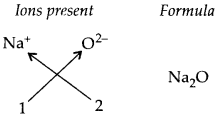Aluminium chloride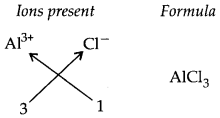Sodium sulphide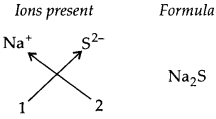Magnesium hydroxide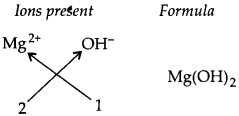Question 8 : Write down the names of compounds represented by the following formulae:

1.   AI2(S04)3

2.   CaCI2

3.   K2S04

4.   KNO3

5.   CaCO3

(i) Aluminium sulphate
(ii) Calcium chloride
(iii) Potassium sulphate
(iv) Potassium nitrate
(v) Calcium carbonate

Question 9 :
What is meant by the term chemical formulae?

The chemical formula of a compound is a symbolic representation of its composition. e.g., formula of calcium oxide.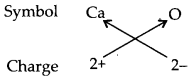Thus, chemical formula of calcium oxide is CaO.

Question 10 : How many atoms are present in a
(i) H2S molecule and
(ii)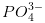ion?

Answer 10 : (i) 3 atoms because H2S molecule has two atoms of hydrogen and one atom ofsulphur.
(ii) 5 atoms becauseion has one atom of phosphorus and four atoms ofoxygen.

Todays Deals### Chapter 3 Atoms And Molecules Contributorskrishan

Name:
Email:

# Latest News# 9000 interview questions in different categories